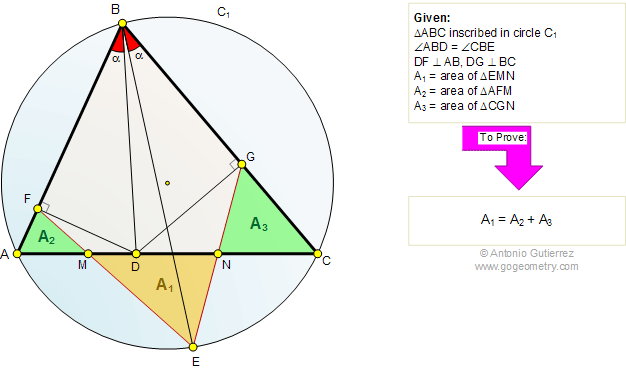# Problem 532: Triangle, Circumcircle, Equal Angles, Perpendicular, Area. Level: High School, SAT, College Geometry

 The figure shows a triangle ABC inscribed in a circle C1. The angles ABD and CBE are congruent. DF is perpendicular to AB and DG is perpendicular to BC. If A1, A2, and A3 are the areas of triangles EMN, AFM, and CGN, respectively, prove that A1 = A2 + A3.Home | Search | Geometry | Problems | All Problems | Visual Index | 531-540 | Email | Post a comment or solution | By Antonio Gutierrez